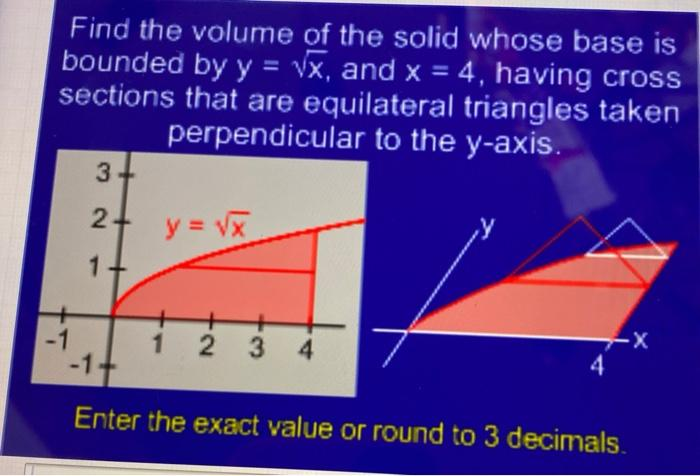### Create an Account

Home / Questions / Find the volume of the solid whose base is bounded by y = vx, and x = 4, having cross sect...

# Find the volume of the solid whose base is bounded by y = vx, and x = 4, having cross sections that are equilateral triangles taken perpendicular to the y-axis. 3 2 y= Vx 1+ 1 2 3 4 7-X Enter the exac

Find the volume of the solid whose base is bounded by y = vx, and x = 4, having cross sections that are equilateral triangles taken perpendicular to the y-axis. 3 2 y= Vx 1+ 1 2 3 4 7-X Enter the exact value or round to 3 decimals.May 13 2021 View more View LessSubscribe To Get Solution